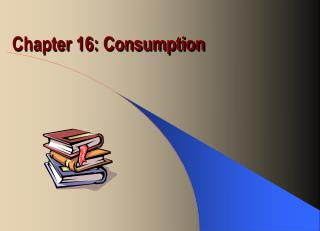DownloadDownload PresentationChapter 16: Consumption

# Chapter 16: Consumption

Télécharger la présentation## Chapter 16: Consumption

- - - - - - - - - - - - - - - - - - - - - - - - - - - E N D - - - - - - - - - - - - - - - - - - - - - - - - - - -
##### Presentation Transcript

1. Chapter 16: Consumption

2. John M. Keynes: Absolute Income Hypothesis • Consumption is a linear function of disposable personal income, C = C + cY C = consumption expenditure Y = disposable income C = autonomous consumption (intercept of the line) c = marginal propensity to consume (slope of the line)

3. Properties of Consumption Function • Consumption is determined by current income • Marginal propensity to consume (MPC = ΔC/ΔY) is between zero and one (0<c<1) • Average propensity to consume (APC = C/Y) falls as income rises

4. Short-run Consumption Function Consumption expenditure C = C + cY Constant APC c C Disposable income

5. Empirical Evidence • High income families have a higher marginal propensity to save (MPS = 1 – MPC) • High income families have a higher average propensity to save (APS = 1 – APC); APC falls with the level of income • In the long-run, autonomous consumption falls to zero (C = 0)

6. Long-run Consumption Function Consumption expenditure C = ćY Variable APC; Ĉ = 0 ć Ĉ Disposable income

7. Irving Fisher: Intertemporal Choice • Consumption decisions are based on current and future income • Current period income = current income plus present value of future income: Y1 + Y2 / (1 + r), where r is a discount rate • Future period income = future income plus future value of current income: Y2 + (1 + r)Y1

8. The Intertemporal Budget Line Future Period B C2 A Y2 C2 C C1 Y1 C1 Current Period

9. The Intertemporal Budget Line • Along BC, there is a trade-off between current and future consumption spending • Along AB, C1<Y1, but C2>Y2: consumers would save in current period to finance consumption in second period • Along AC, C1>Y1, but C2<Y2: consumers would borrow in current period and will pay off debt in future period

10. Consumer Preferences • Consumer preferences are shown by a family of indifference curves • Any combination of current and future consumption along an indifference curve provides the same level of satisfaction for the consumer • A higher indifference curve yields combinations with greater satisfaction

11. Consumer Preferences Future Period Combination B is preferred to combination A because it yields more in both periods B A Current Period

12. The Consumer’s Optimum • Consumer equilibrium is achieved at the tangency of the highest attainable indifference curve and the budget line • The tangency determines the optimum allocation of consumption spending in both periods; i.e. highest level of satisfaction within the budget

13. The Consumer’s Optimum Future Period Higher income shifts the budget line up, positioning the consumer on a higher indifference curve and consumer’s optimum B C2f A C1f C1c C2c Current Period

14. Franco Modigliani: Life Cycle Hypothesis • Consumption depends on income and wealth C = Consumption expenditure W = Consumer wealth R = Length of productive life time T = Years of life

15. Consumption Function C = (W + RY)/T = (1/T)W + (R/T)Y Define: α = 1/T is the MPC out of wealth β = R/T is the MPC out of income C = αW + βY

16. Consumption Function Consumption expenditure C = αW + βY β 1 αW Disposable income For the United States, α = 0.02 and β = 0.60.

17. Consumption Function Consumption expenditure αW2 αW1 Disposable income Increased wealth shifts the consumption function upward.

18. Consumer Behavior over Life Time • Consumption spending is a stable function of income • Consumers save their leftover income • Consumers accumulate wealth during the productive lifetime • Consumer finance retirement by dissaving and selling-off their assets

19. Consumer Behavior over Life Time \$ Wealth Income Saving Consumption Dissaving R T Years

20. Milton Friedman: Permanent Income Hypothesis • Measured income consists of permanent and transitory income; Y = YP + YT • Permanent income is the average income we make during years of productive life • Transitory income is the random variation from the average

21. Consumption Function • Consumption is a function of permanent income C = αYP • Consumers use saving and borrowing to smooth consumption in response to transitory changes in income

22. Determinants of Consumption • Combining all the theories, we can conclude that consumption depends on Current income Expected future income Wealth Interest rate